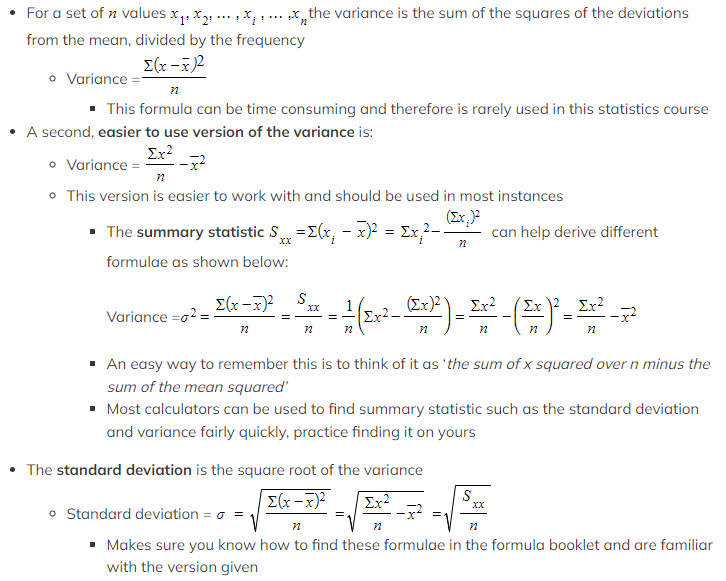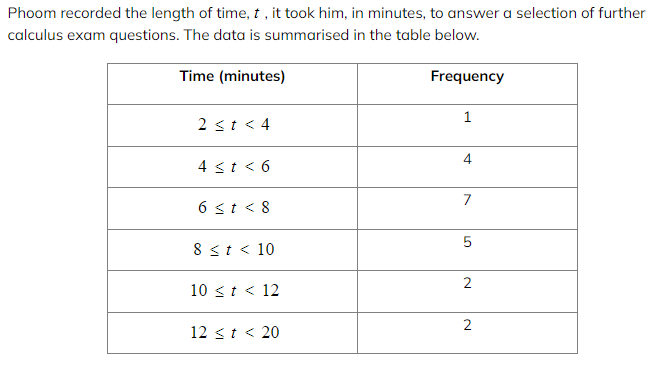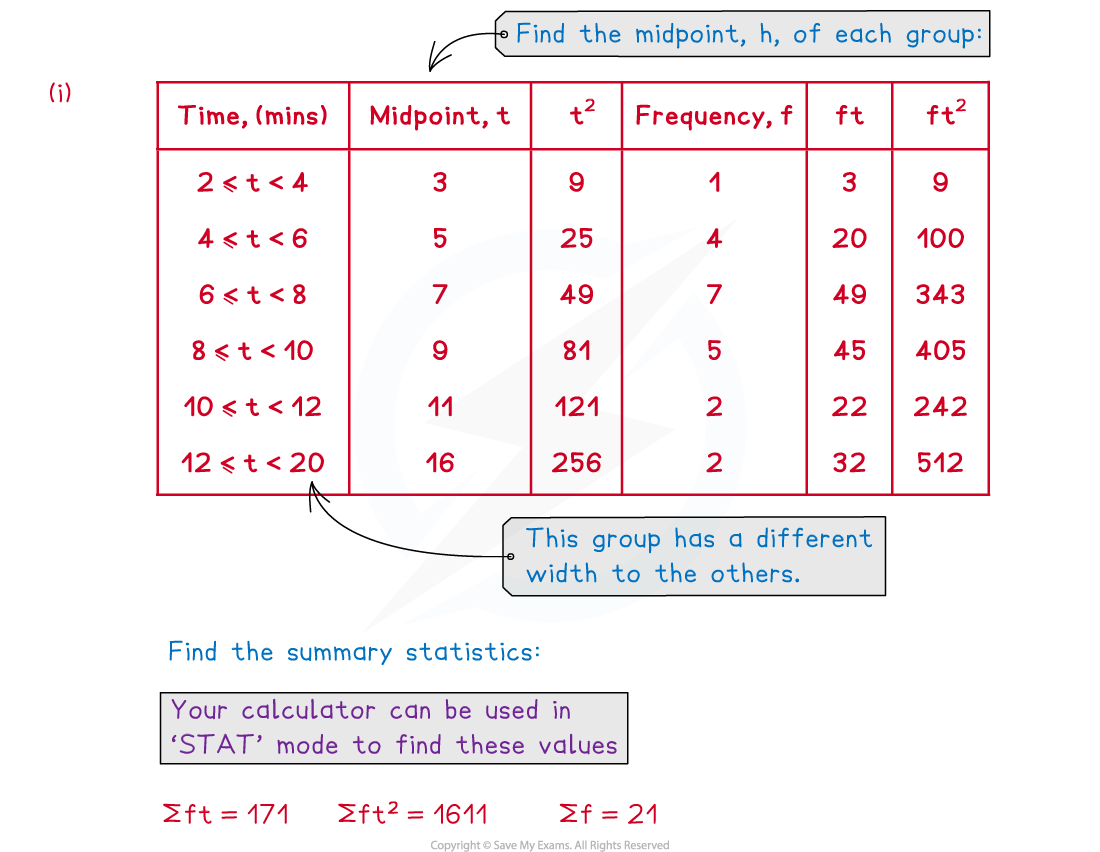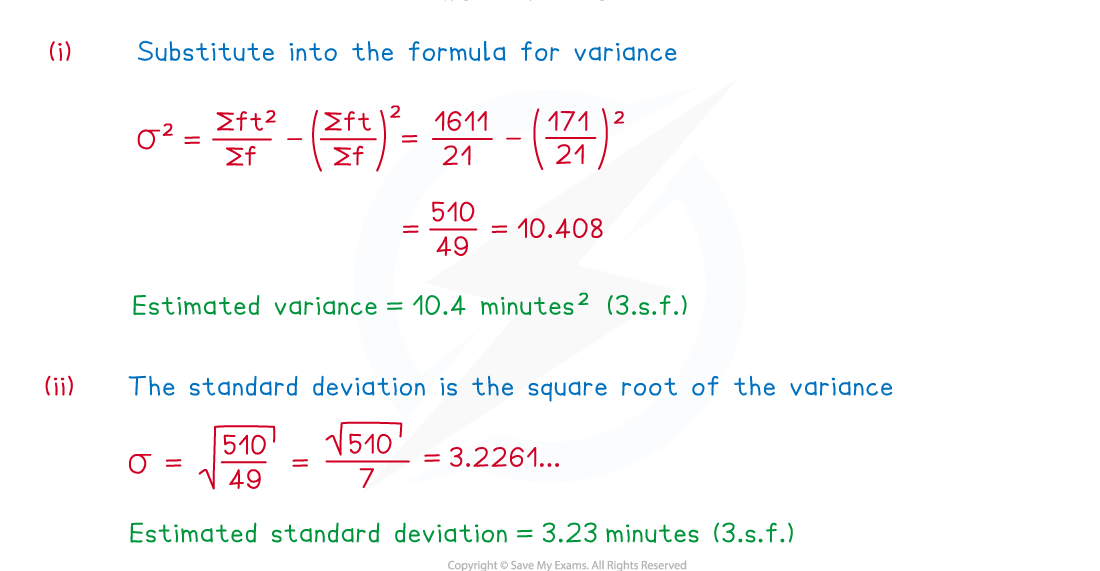# AQA A Level Maths: Statistics复习笔记2.1.3 Standard Deviation & Variance

### Standard Deviation & Variance

The variance is another measure for the spread of the data, it measures the variability from the mean of the data.

#### What is the variance and the standard deviation?

• The variance is a statistic that tells us how varied a set of data is
• Data that is more spread out will have a greater variance
• Data that is consistent and close together will have a smaller variance
• The standard deviation is the square root of the variance
• The symbol for the population standard deviation is the lowercase Greek letter sigma, σ  and for variance is sigma squared, σ2
• Standard deviation and variance are used interchangeably within this course so make sure you look out for which one a question shows or asks for

#### How are the variance and standard deviation calculated?

• There is more than one formula that can be used for calculating the variance, and you should choose the most useful one
•Makes sure you know how to find these formulae in the formula booklet and are familiar with the version given
• The units for standard deviation are the same as the units for the data and the units for variance are the same as the units for the data but squared

#### How are the variance and standard deviation calculated from a frequency table?

• The method for finding the variance from a frequency table is similar to that of the mean#### Worked Example(i)Calculate an estimate of the variance of the time taken to complete a question, include units with your answer.
(ii)Write down an estimate of the standard deviation of the time taken to complete a question, include units with your answer.#### Exam Tip

• Look out for whether a question gives or asks for the standard deviation or variance, especially if the question is using sigma notation.
• Choose which formula to use wisely, most of the time the summary statistics will be given so only one of the formulae will be possible. On the rare occasion that you are asked to calculate directly from a table think carefully about which version of the formula is quickest and easiest to use. It will almost always be the second version given in this revision note.